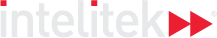### Course Overview

Trigonometry 2 explores how to work with the various trigonometric functions and equations. The laws of sines and cosines are introduced, along with their practical applications. The course concludes with a look at inverse functions and how to solve for missing values like sides or angles in triangles.

HOURS OF INSTRUCTION: 15

### Course Outline

• Sum and Difference of Trigonometric Functions
• Trigonometric Identities and Equations
• Laws of Trigonometric Functions and Area of Triangles
• Inverse of Trigonometric Functions
• Solution of Triangles